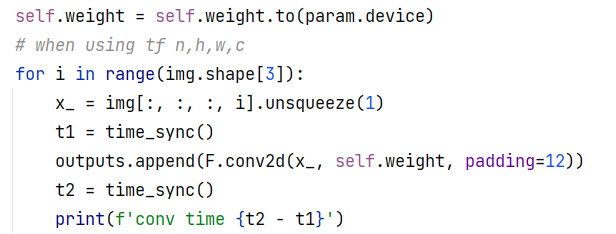# Calculation time of 'torch.nn.functional.conv2d '

Why is it so time-consuming！！！
I need to use custom parameters in the network. So I used this function in forward propagation, but practice shows that its calculation is very time-consuming! Before the calculation, I have placed the input and weight on the GPU, but the time is as high as 2S. I don’t know why. Can someone help me?this code is integrated in yolov5, the img shape is [16,640,640,3], the x_ shape is [16,1,640,640], the weight shape is [1,1,25,25].

As can be seen from the figure, the time is up to 2 ~ 3 seconds.

Depending on the hardware (GPU) used this isn’t entirely unexpected. Keep in mind that scaling is basically quadratic with respect to the spatial dimension, with `(640**2)/(224**2)` being about 8x.

However, indexing the channel dimensions of the image seems highly unusual (what is the purpose of this?) and would also slow things down due to the multiple kernel invocations.

What is the GPU in this setup and are there any other optimizations being applied (e.g., fp16)?

Thank you for your reply. I did an independent test later: in the same device, when I randomly initialize the input and weight of the same dimension for operation, the time is greatly reduced, and I don’t know what the problem is. Is it because of my integration in yolov5?
This is an attempt of depth-wise convolution.
GPU settings are the same as yolov5.

GPU settings are the same as yolov5.
I assume that means you are using V100?

How is `img` initialized in this setup? It would be very helpful if you could post a runnable code snippet that reproduces the problem (e.g., make a dummy conv2d layer that has the same shapes) for debugging.